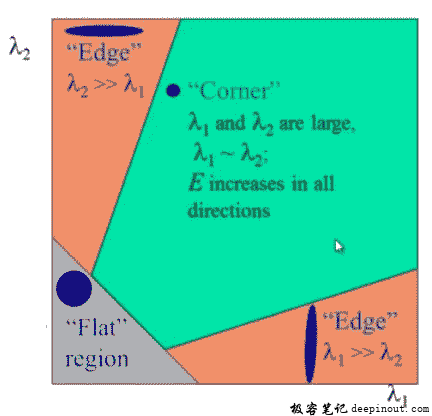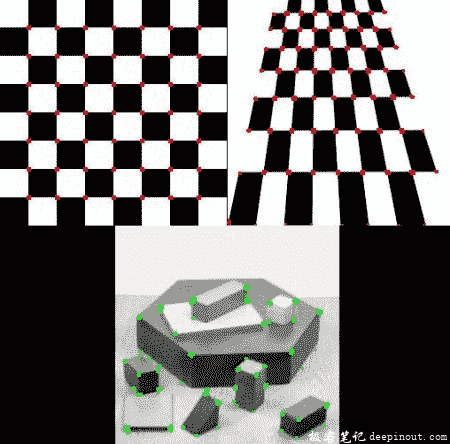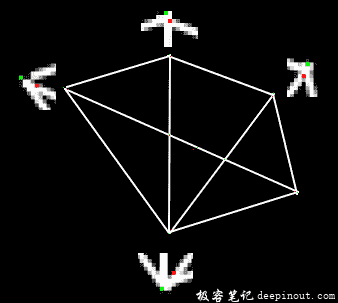# OpenCV-Python Harris 角点检测

## Harris 角点检测器理论

E(u,v) = \sum_ {x,y} \underbrace{w(x,y)} _ {window function} [\underbrace{I(x+u,y+v)} _ {shifted intensity}-\underbrace{I(x,y)} _ {intensity}] ^ 2

E(u,v) \approx \begin {bmatrix} u&v \end {bmatrix} M \begin {bmatrix} u\\v \end {bmatrix}

M = \sum_ {x,y} w(x,y)\begin{bmatrix} I_x I_x&I_x I_y\\I_x I_y&I_y I_y \end{bmatrix}

R = det(M)-k(trace(M))^ 2

• det(M)=\lambda_1 \lambda_2
• trace(M)= \lambda_1+\lambda_2
• λ1和λ2是 M 的特征值

• 当λ1和λ2都很小时，|R|也很小，该区域是平面。
• 当λ1>>λ2 或者R<0时，R该区域是边缘。
• 当 λ1 和λ2大且λ1∼λ2时，R很大，该区域是一个角点。## OpenCV 中的 Harris 角点检测器

OpenCV 中的 cv.cornerHarris() 函数用来实现 Harris 角点检测。它的参数是：

• img – 输入图像，应为 float32 类型的灰度图。
• blockSize – 角点检测所考虑的邻域大小。
• ksize – Sobel 导数的内核大小。
• k – Harris 检测器方程中的自由参数。

import numpy as np
import cv2 as cv
filename = 'chessboard.png'
gray = cv.cvtColor(img,cv.COLOR_BGR2GRAY)
gray = np.float32(gray)
dst = cv.cornerHarris(gray,2,3,0.04)
#result is dilated for marking the corners, not important
dst = cv.dilate(dst,None)
# Threshold for an optimal value, it may vary depending on the image.
img[dst>0.01*dst.max()]=[0,0,255]
cv.imshow('dst',img)
if cv.waitKey(0) & 0xff == 27:
cv.destroyAllWindows()## 具有亚像素级精度的角点

import numpy as np
import cv2 as cv
filename = 'chessboard2.jpg'
gray = cv.cvtColor(img,cv.COLOR_BGR2GRAY)
# find Harris corners
gray = np.float32(gray)
dst = cv.cornerHarris(gray,2,3,0.04)
dst = cv.dilate(dst,None)
ret, dst = cv.threshold(dst,0.01*dst.max(),255,0)
dst = np.uint8(dst)
# find centroids
ret, labels, stats, centroids = cv.connectedComponentsWithStats(dst)
# define the criteria to stop and refine the corners
criteria = (cv.TERM_CRITERIA_EPS + cv.TERM_CRITERIA_MAX_ITER, 100, 0.001)
corners = cv.cornerSubPix(gray,np.float32(centroids),(5,5),(-1,-1),criteria)
# Now draw them
res = np.hstack((centroids,corners))
res = np.int0(res)
img[res[:,1],res[:,0]]=[0,0,255]
img[res[:,3],res[:,2]] = [0,255,0]
cv.imwrite('subpixel5.png',img)• 回顶